# NCERT solutions for Class 10 Maths chapter 2 - Polynomials [Latest edition]

#### Chapters## Solutions for Chapter 2: Polynomials

Below listed, you can find solutions for Chapter 2 of CBSE NCERT for Class 10 Maths.

Exercise 2.1Exercise 2.2Exercise 2.3Exercise 2.4
Exercise 2.1 [Page 28]

### NCERT solutions for Class 10 Maths Chapter 2 Polynomials Exercise 2.1 [Page 28]

Exercise 2.1 | Q 1.1 | Page 28

The graphs of y = p(x) are given in following figure, for some polynomials p(x). Find the number of zeroes of p(x), in the following.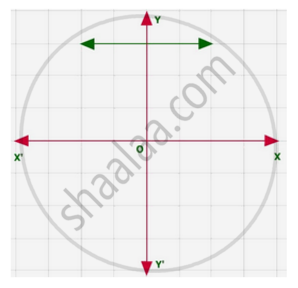Exercise 2.1 | Q 1.2 | Page 28

The graphs of y = p(x) are given in following figure, for some polynomials p(x). Find the number of zeroes of p(x)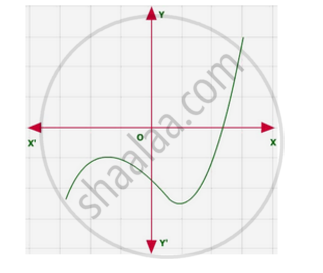Exercise 2.1 | Q 1.3 | Page 28

The graphs of y = p(x) are given in following figure, for some polynomials p(x). Find the number of zeroes of p(x)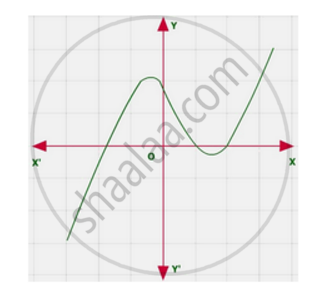Exercise 2.1 | Q 1.4 | Page 28

The graphs of y = p(x) are given in following figure, for some polynomials p(x). Find the number of zeroes of p(x)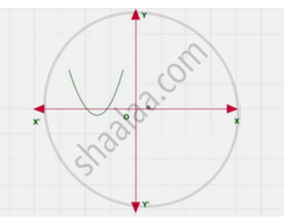Exercise 2.1 | Q 1.5 | Page 28

The graphs of y = p(x) are given in following figure, for some polynomials p(x). Find the number of zeroes of p(x)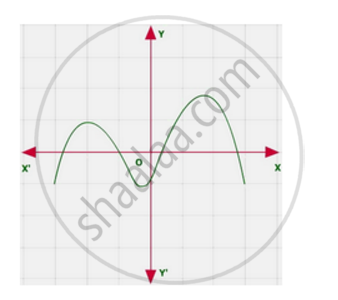Exercise 2.1 | Q 1.6 | Page 28

The graphs of y = p(x) are given in following figure, for some polynomials p(x). Find the number of zeroes of p(x)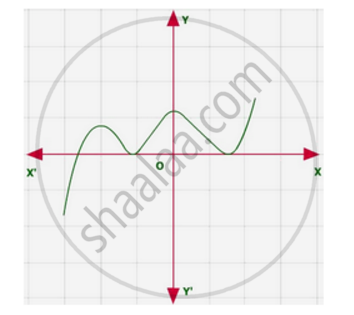Exercise 2.2 [Page 33]

### NCERT solutions for Class 10 Maths Chapter 2 Polynomials Exercise 2.2 [Page 33]

Exercise 2.2 | Q 1.1 | Page 33

Find the zeroes of the following quadratic polynomials and verify the relationship between the zeroes and the coefficients

x2 – 2x – 8

Exercise 2.2 | Q 1.2 | Page 33

Find the zeroes of the following quadratic polynomials and verify the relationship between the zeroes and the coefficients

4s2 – 4s + 1

Exercise 2.2 | Q 1.3 | Page 33

Find the zeroes of the following quadratic polynomials and verify the relationship between the zeroes and the coefficients.

6x2 – 3 – 7x

Exercise 2.2 | Q 1.4 | Page 33

Find the zeroes of the following quadratic polynomials and verify the relationship between the zeroes and the coefficients

4u2 + 8u

Exercise 2.2 | Q 1.5 | Page 33

Find the zeroes of the following quadratic polynomials and verify the relationship between the zeroes and the coefficients

t2 – 15

Exercise 2.2 | Q 1.6 | Page 33

Find the zeroes of the following quadratic polynomials and verify the relationship between the zeroes and the coefficients

3x2 – x – 4

Exercise 2.2 | Q 2.1 | Page 33

Find a quadratic polynomial each with the given numbers as the sum and product of its zeroes respectively

1/4 , -1

Exercise 2.2 | Q 2.2 | Page 33

Find a quadratic polynomial each with the given numbers as the sum and product of its zeroes respectively.

sqrt2 , 1/3

Exercise 2.2 | Q 2.3 | Page 33

Find a quadratic polynomial each with the given numbers as the sum and product of its zeroes respectively

0, sqrt5

Exercise 2.2 | Q 2.4 | Page 33

Find a quadratic polynomial each with the given numbers as the sum and product of its zeroes respectively

1, 1

Exercise 2.2 | Q 2.5 | Page 33

Find a quadratic polynomial each with the given numbers as the sum and product of its zeroes respectively

-1/4 ,1/4

Exercise 2.2 | Q 2.6 | Page 33

Find a quadratic polynomial each with the given numbers as the sum and product of its zeroes respectively

4, 1

Exercise 2.3 [Page 36]

### NCERT solutions for Class 10 Maths Chapter 2 Polynomials Exercise 2.3 [Page 36]

Exercise 2.3 | Q 1.1 | Page 36

Divide the polynomial p(x) by the polynomial g(x) and find the quotient and remainder in each of the following

p(x) = x3 – 3x2 + 5x – 3, g(x) = x2 – 2

Exercise 2.3 | Q 1.2 | Page 36

Divide the polynomial p(x) by the polynomial g(x) and find the quotient and remainder in each of the following : p(x) = x4 – 3x2 + 4x + 5,   g(x) = x2 + 1 – x

Exercise 2.3 | Q 1.3 | Page 36

Divide the polynomial p(x) by the polynomial g(x) and find the quotient and remainder in each of the following

p(x) = x4 – 5x + 6, g(x) = 2 – x2

Exercise 2.3 | Q 2.1 | Page 36

Check whether the first polynomial is a factor of the second polynomial by dividing the second polynomial by the first polynomial

t2 – 3, 2t4 + 3t3 – 2t2 – 9t – 12

Exercise 2.3 | Q 2.2 | Page 36

Check whether the first polynomial is a factor of the second polynomial by dividing the second polynomial by the first polynomial

x2 + 3x + 1, 3x4 + 5x3 – 7x2 + 2x + 2

Exercise 2.3 | Q 2.3 | Page 36

Check whether the first polynomial is a factor of the second polynomial by dividing the second polynomial by the first polynomial

x3 – 3x + 1, x5 – 4x3 + x2 + 3x + 1

Exercise 2.3 | Q 3 | Page 36

Obtain all other zeroes of 3x4 + 6x3 – 2x2 – 10x – 5, if two of its zeroes are sqrt(5/3) and - sqrt(5/3)

Exercise 2.3 | Q 4 | Page 36

On dividing x3 – 3x2 + x + 2 by a polynomial g(x), the quotient and remainder were x – 2 and –2x + 4, respectively. Find g(x)

Exercise 2.3 | Q 5.1 | Page 36

Give examples of polynomials p(x), g(x), q(x) and r(x), which satisfy the division algorithm

deg p(x) = deg q(x)

Exercise 2.3 | Q 5.2 | Page 36

Give examples of polynomials p(x), g(x), q(x) and r(x), which satisfy the division algorithm

deg q(x) = deg r(x)

Exercise 2.3 | Q 5.3 | Page 36

Give examples of polynomials p(x), g(x), q(x) and r(x), which satisfy the division algorithm

deg r(x) = 0

Exercise 2.4 [Pages 36 - 37]

### NCERT solutions for Class 10 Maths Chapter 2 Polynomials Exercise 2.4 [Pages 36 - 37]

Exercise 2.4 | Q 1.1 | Page 36

Verify that the numbers given along side of the cubic polynomials are their zeroes. Also verify the relationship between the zeroes and the coefficients.

2x^3 + x^2 – 5x + 2 ; 1/2, 1, – 2

Exercise 2.4 | Q 1.2 | Page 36

Verify that the numbers given alongside of the cubic polynomials below are their zeroes. Also verify the relationship between the zeroes and the coefficients in each case

x3 – 4x2 + 5x – 2; 2, 1, 1

Exercise 2.4 | Q 2 | Page 36

Find a cubic polynomial with the sum, sum of the product of its zeroes taken two at a time, and the product of its zeroes as 2, − 7, − 14 respectively

Exercise 2.4 | Q 3 | Page 37

If the zeroes of the polynomial x3 – 3x2 + x + 1 are a – b, a, a + b, find a and b

Exercise 2.4 | Q 4 | Page 37

If two zeroes of the polynomial x4 – 6x3 – 26x2 + 138x – 35 are 2 ± sqrt3 , find other zeroes

Exercise 2.4 | Q 5 | Page 37

If the polynomial x4 – 6x3 + 16x2 – 25x + 10 is divided by another polynomial x2 – 2x + k, the remainder comes out to be x + a, find k and a.

## Solutions for Chapter 2: Polynomials

Exercise 2.1Exercise 2.2Exercise 2.3Exercise 2.4## NCERT solutions for Class 10 Maths chapter 2 - Polynomials

Shaalaa.com has the CBSE Mathematics Class 10 Maths CBSE solutions in a manner that help students grasp basic concepts better and faster. The detailed, step-by-step solutions will help you understand the concepts better and clarify any confusion. NCERT solutions for Mathematics Class 10 Maths CBSE 2 (Polynomials) include all questions with answers and detailed explanations. This will clear students' doubts about questions and improve their application skills while preparing for board exams.

Further, we at Shaalaa.com provide such solutions so students can prepare for written exams. NCERT textbook solutions can be a core help for self-study and provide excellent self-help guidance for students.

Concepts covered in Class 10 Maths chapter 2 Polynomials are Geometrical Meaning of the Zeroes of a Polynomial, Relationship Between Zeroes and Coefficients of a Polynomial, Division Algorithm for Polynomials, Polynomials, Polynomials, Geometrical Meaning of the Zeroes of a Polynomial, Relationship Between Zeroes and Coefficients of a Polynomial, Division Algorithm for Polynomials, Polynomials, Polynomials.

Using NCERT Class 10 Maths solutions Polynomials exercise by students is an easy way to prepare for the exams, as they involve solutions arranged chapter-wise and also page-wise. The questions involved in NCERT Solutions are essential questions that can be asked in the final exam. Maximum CBSE Class 10 Maths students prefer NCERT Textbook Solutions to score more in exams.

Get the free view of Chapter 2, Polynomials Class 10 Maths additional questions for Mathematics Class 10 Maths CBSE, and you can use Shaalaa.com to keep it handy for your exam preparation.